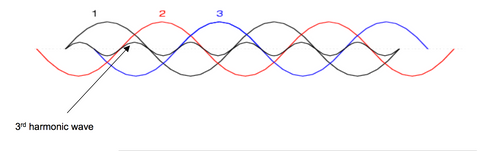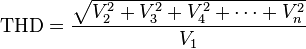# Harmonics (electrical power)

Harmonic

Voltages and currents in an electric power system are a result of non-linear electric loads. Harmonic frequencies in the power grid are a frequent cause of power quality problems. Harmonics in power systems result in increased heating in the equipment and conductors, misfiring in variable speed drives, and torque pulsations in motors. Reduction of harmonics is considered desirable.

Current harmonics

In a normal alternating current power system, the current varies sinusoidally at a specific frequency, usually 50 or 60 hertz. When a linear electrical load is connected to the system, it draws a sinusoidal current at the same frequency as the voltage (though usually not in phase with the voltage).

Current harmonics are caused by non-linear loads. When a non-linear load, such as a rectifier, is connected to the system, it draws a current that is not necessarily sinusoidal. The current waveform can become quite complex, depending on the type of load and its interaction with other components of the system. Regardless of how complex the current waveform becomes, as described through Fourier series analysis, it is possible to decompose it into a series of simple sinusoids, which start at the power system fundamental frequency and occur at integer multiples of the fundamental frequency.

Further examples of non-linear loads include common office equipment such as computers and printers, Fluorescent lighting, battery chargers and also variable-speed drives.

Third-order harmonics

In power systems, Harmonics are multiples of the fundamental wavelength. Thus, the third order harmonic is the third multiple of the fundamental wavelength. This type of harmonics is generated in non-linear loads. Examples of nonlinear loads include transistors, electrical motors, and the non-ideal transformer. Nonlinear loads create disturbances in the fundamental harmonic, which produce all types of harmonics. However, in this section we focus on the 3rd order harmonic due to its certain special characteristics in the context of powers systems.Our power is supplied by a three phase system, where each phase is 120 degrees apart. This is done for two reasons: Firstly it is because generators/motors that use three phases are more efficient due to the constant torque the phases supply, and secondly it is because after power is supplied to a load, the three phases can theoretically be added onto a neutral wire and cancel each other out. This saves the utility from creating return wiring to the power plant. However, if the 3 phases contain 3rd order harmonics, the currents will not fully add to zero. As seen in the figure, the 3rd harmonic will add constructively with the 3rd harmonics within the other phases. This leads to an oscillating current in the neutral wire, which can be dangerous since it is designed to carry minimal current.To avoid 3rd harmonics adding together Delta connections are used, and the current is cycled around the connection instead of combining into the neutral of a Wye connection.

compact fluorescent lamp is one example of an electrical load with a non-linear characteristic, due to therectifier circuit it uses. The current waveform, blue, is highly distorted.

Voltage harmonics

Voltage harmonics are mostly caused by current harmonics. The voltage provided by the voltage source will be distorted by current harmonics due to source impedance. If the source impedance of the voltage source is small, current harmonics will cause only small voltage harmonics.

Harmonics fundamentals

Harmonics provides a mathematical analysis of distortions to a current or voltage waveform. Based on Fourier series, harmonics can describe any periodic wave as a summation of simple sinusoidal waves which are integer multiples of the fundamental frequency.

Harmonics are steady-state distortions to current and voltage waves and repeat every cycle. They are different from transient distortions to power systems such as spikes, dips and impulses.

Total harmonic distortion

Total harmonic distortion, or THD is a common measurement of the level of harmonic distortion present in power systems. THD is defined as the ratio of total harmonics to the value at fundamental frequency.where Vn is the RMS voltage of nth harmonic and n = 1 is the fundamental frequency.

Effects

One of the major effects of power system harmonics is to increase the current in the system. This is particularly the case for the third harmonic, which causes a sharp increase in the zero sequence current, and therefore increases the current in the neutral conductor. This effect can require special consideration in the design of an electric system to serve non-linear loads.

In addition to the increased line current, different pieces of electrical equipment can suffer effects from harmonics on the power system.

Motors

Electric motors experience losses due to hysteresis and losses due to eddy currents set up in the iron core of the motor. These are proportional to the frequency of the current. Since the harmonics are at higher frequencies, they produce higher core losses in a motor than the power frequency would. This results in increased heating of the motor core, which (if excessive) can shorten the life of the motor. The 5th harmonic causes a CEMF (counter electromotive force) in large motors which acts in the opposite direction of rotation. The CEMF is not large enough to counteract the rotation, however it does play a small role in the resulting rotating speed of the motor.

Telephones

In the United States, common telephone lines are designed to transmit frequencies between 300 and 3400 Hz. Since electric power in the United States is distributed at 60 Hz, it normally does not interfere with telephone communications because its frequency is too low.

## Sources

A pure sinusoidal voltage is a conceptual quantity produced by an ideal AC generator built with finely distributed stator and field windings that operate in a uniform magnetic field. Since neither the winding distribution nor the magnetic field are uniform in a working AC machine, voltage waveform distortions are created, and the voltage-time relationship deviates from the pure sine function. The distortion at the point of generation is very small (about 1% to 2%), but nonetheless it exists. Because this is a deviation from a pure sine wave, the deviation is in the form of a periodic function, and by definition, the voltage distortion contains harmonics.

When a sinusoidal voltage is applied to a certain type of load, the current drawn by the load is determined by the voltage and impedance and follows the voltage waveform. These loads are referred to as linear loads; examples of linear loads are resistive heaters, incandescent lamps, and constant speed induction and synchronous motors.

In contrast, some loads cause the current to vary disproportionately with the voltage during each cyclic period. These are classified as nonlinear loads, and the current taken by them has a nonsinusoidal waveform.

When there is significant impedance in the path from the power source to a nonlinear load, these current distortions will also produce distortions in the voltage waveform at the load. However, in most cases where the power delivery system is functioning correctly under normal conditions, the voltage distortions will be quite small and can usually be ignored.

Waveform distortion can be mathematically analysed to show that it is equivalent to superimposing additional frequency components onto a pure sinewave. These frequencies are harmonics (integer multiples) of the fundamental frequency, and can sometimes propagate outwards from nonlinear loads, causing problems elsewhere on the power system.

The classic example of a non-linear load is a rectifier with a capacitor input filter, where the rectifier diode only allows current to pass to the load during the time that the applied voltage exceeds the voltage stored in the capacitor, which might be a relatively small portion of the incoming voltage cycle.

Other examples of nonlinear loads are battery chargers, electronic ballasts, variable frequency drives, and switching mode power supplies.

### 3JIndustry Specialized Distributor - Buy & Sales Used Products

Buy, Sell, Used Drive, Plc, Automation, Relay, Plc, Power Supply, Sensor, Timer, Contactor, Breaker, Cable, Touchscreen, Omron, Moeller, Eaton, AB.

• 3J Industry